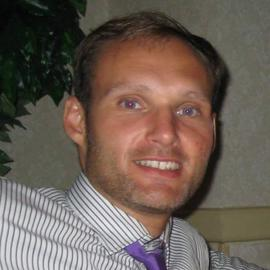# Biography

I am currently an associate professor in the Department of Mathematics at the University of Utah. My main focus of research is in probability theory, and within that I study two-dimensional conformally invariant systems. The basic model of these are the Schramm-Loewner Evolution and its variants. I also have interests in statistical mechanics, random walks in random environments, directed polymer models, last passage percolation, and random matrix theory.

Interests
• Probability Theory
• Stochastic Analysis
• 2D Conformally Invariant Systems
• Directed Polymer Models
• Last Passage Percolation
• Random Matrices
Education
• PhD in Mathematics, 2008

Courant Institute of Mathematical Sciences at New York University

• BSc in Mathematics, 2002

University of Alberta

# Contact Information

• lastname (at) math (dot) utah (dot) edu
• 801-585-1643
• 155 S 1400 E Room 233, Salt Lake City, UT 84112-0090
• LCB 114

# Recent Publications

Dimension Results for the Spectral Measure of the Circular Beta Ensembles.
Annals of Applied Probability, 32, 4642–4680. (2022).
The Green's function of the parabolic Anderson model and the continuum directed polymer.
arXiv:2208.11255 [math.PR] . (2022).
On the passage time geometry of the last passage percolation problem.
ALEA Lat. Am. J. Probab. Math. Stat., 18, 211–247. (2021).
Pole dynamics and an integral of motion for multiple SLE(0).
arXiv:2011.05714 [math.CV] . (2020).
Busemann functions and semi-infinite O'Connell-Yor polymers.
Bernoulli, 26, 1927–1955. (2020).

# Recent & Upcoming Talks

The Fundamental Solution for the 1+1-dimensional Stochastic Heat Equation
Conformal Field Theory for Multiple SLEs
Loewner Dynamics for the Multiple SLE(0) Process
Random Geometry in the Spectral Measure of the Circular Beta Ensemble
Random Matrix Theory for Homogenization of Composites